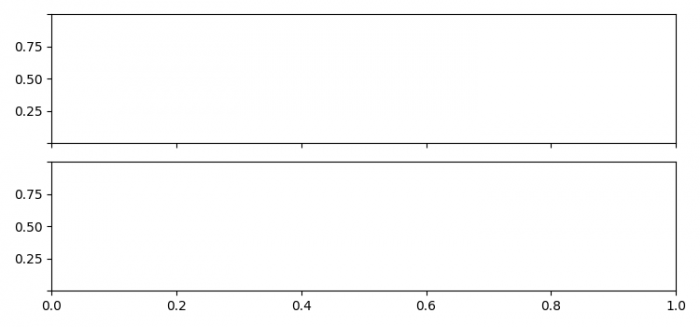# How to remove the first and last ticks label of each Y-axis subplot in Matplotlib?

To remove the first and last ticks label of each Y-axis subplot, we can take the following steps −

• Set the figure size and adjust the padding between and around the subplots.

• Create a figure and a set of subplots.

• Iterate the axes and set the first and last ticklabel's visible=False.

• To display the figure, use show() method.

## Example

import matplotlib.pyplot as plt

plt.rcParams["figure.figsize"] = [7.50, 3.50]
plt.rcParams["figure.autolayout"] = True

fig, ax = plt.subplots(2, sharex=True)

for a in ax:
plt.setp(a.get_yticklabels(), visible=False)
plt.setp(a.get_yticklabels()[-1], visible=False)

plt.show()

## Output